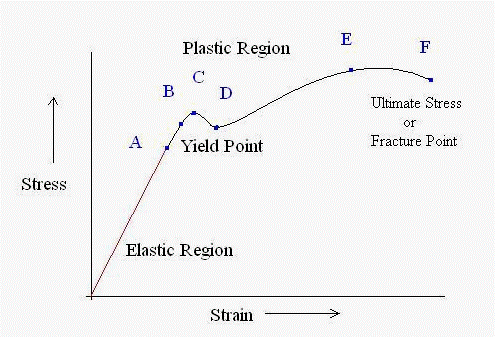# What is Proof Stress? Significance and Uses

The term stress is a mechanical property of any kind of object which indicates internal resistance offered by it under any kind of deformation. Consequently, from various mechanical properties, stress is an important characteristic while selecting materials. In a broad sense, proof stress is the amount of stress at which a material undergoes plastic deformation. It is not exactly the yield stress. But it is the offset yield stress.

# What is Proof Stress?

Therefore, the proof stress specifically means the point of deformation where the subjected material cannot get back to its original state. We might understand the term if we dig deep into the stress-strain diagram.Figure- 1: Stress-strain diagram

The figure above (Figure- 1) is from a laboratory tension test of a specimen. Firstly, the jaws of a testing machine gripped the specimen. The tester should observe the gauge lengths. simultaneously. Then the data were plotted on a graph with the ordinate representing the stress (load/area) and the abscissa representing the strain (elongation/length).

From origin to point A is the Elastic Region where the stress is unable to cause any kind of malformation as the specimen retains its original form. Point B is the Elastic limit at which the specimen will not return to its original state in the time of unloading and point C is Yield Point where there is an appreciable elongation without any corresponding increase of load. That implies that the object or specimen totally deformed at yield point. This is the phenomena of plastic deformation. And this level of stress where the specimen achieve plastic deformity is the Proof Stress.

Probably the reason of addressing this as offset yield stress because it is the required stress to make the material yield. It may make confusion between the proof stress and the ultimate stress. While proof stress causes permanent material deformation whereas, ultimate stress is the maximum stress that a material can stand before it fails. Proof stress of material not only stretches the bonds but also breaks them. This causes dislocation of atoms which is predominantly the reason behind permanent deformation.

## Uses of Proof stress

Considering the engineering perspective, proof stress helps the material selection process. For instance, steel in the beams can only handle a certain amount of stress before it yields. By determining and analyzing various proof stresses of materials, suitable ones can be easily equipped.

If the material is not allowed to be distorted, the stresses in the application should not exceed the proof stress limit.

### Disclaimer

Please note that the information in Civiltoday.com is designed to provide general information on the topics presented. The information provided should not be used as a substitute for professional services.